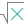#COMBIN (PQL - xl)

This function calculates the number of possible combinations for a specified number of items

• Library: PQL \ Spreadsheet \ Math & Trig
• Compatibility: Any content (regardless of data source) in the Tabulate spreadsheet module
• Solve: This function can be used with the Solve plug-in: both as part of the objective function and constraint functions

#### Syntax

COMBIN(number, number chosen)

##### Function Arguments
 Name Description Type Optional number Number representing the number of items; if not an integer, the number is truncated; must be positive and greater than or equal to n Number number chosen Number of items in each possible permutation; if not an integer, the number is truncated; must be positive Number